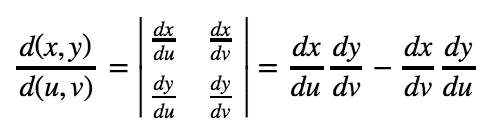# Change in variables

### Change in variables

#### Lessons

Notes:

Transformations

Recall that in Integral Calculus, we can change the variable $x$ to $u$ of an integral using u-substitution. In other words, we can change from

$\int f(x)dx \to \int f(u)du$

We would like to do something similar like this with double integrals.

Transformations is about changing from one variable to another. We will first start by transforming regions.

Jacobian of a Transformation

The Jacobian of a transformation $x=g(u,v)$ & $y=h(u,v)$ is the following:It is the determinant of a 2 x 2 matrix.

Change of Variables for a Double Integral

Suppose we want to integrate the function $f(x,y)$ in the region $R$ under the transformation $x=g(u,v)$ & $y=h(u,v)$. Then the integral will now become:

$\int \int_R f(x,y)dA = \int \int_S f(g(u,v), h(u,v)) \left| \frac{d(x,y)}{d(u,v)}\right| du dv$

• Introduction
Change in Variables Overview:
a)
Transformations
• Transformation = change one variable to another
• Similar to u-substitution in integral calculus
• $xy$-coordinate $\to$ $uv$-coordinate
• An Example of Change in Variable of Regions

b)
Jacobian of a Transformation
• Definition of Jacobian
• Determinant of a 2 x 2 matrix
• Deals with derivatives

c)
Change of Variables for a Double Integral
• All $x$'s & $y$'s become $u$'s & $v$'s
• Extra term is absolute value of Jacobian
• An Example

• 1.
Finding the Transformations
Suppose we have $\, R$, where $\, R \,$ is the region bounded by $\, y = x + 2, y = -x$, and $\, y = \frac{x}{2}$. Use the transformation $\, x = \frac{1}{3}u - \frac{1}{3}v \,$ and $\, y = \frac{1}{3}u + \frac{1}{3}v \,$ to determine the new region.

• 2.
Suppose we have $\, R$, where $\, R \,$ is the region bounded by $\, y = \frac{1}{x}, y = \frac{2}{x}, x = 2, x = 4$. Use the transformation $\, x = 2u \,$ and $\, y = \frac{v}{u} \,$ to determine the new region.

• 3.
Finding the Jacobian
Given that the transformations are $\, x = 2u + 4v^{2} \,$ and $\, y = u^{2} - 4v$, find the Jacobian.

• 4.
Given that the transformations are $\, x = u^{3}v^{5} \,$ and $\, y = \frac{u}{v}$, find the Jacobian.

• 5.
Changing the Variables & Integrating
Evaluate $\, \int\int_{R} x - ydA \,$ where $\,R \,$ is the region bounded by $\, \frac{x^{2}}{4} + \frac{y^{2}}{9} = 1 \,$ using the transformation $\, x = 2v, \,$ and $\, y = 3v$.

• 6.
Evaluate $\, \int\int_{R} 2xydA \,$ where $\,R \,$ is the region bounded by $\, xy = 2, xy = 4, x = 2, x = 4\,$ using the transformation $\, x = 2u, \,$ and $\, y = \frac{v}{u}$.